# Examples Lattice Construction and Lattice Traversal Examples Verification

• Slides: 16Examples Lattice Construction and Lattice Traversal Examples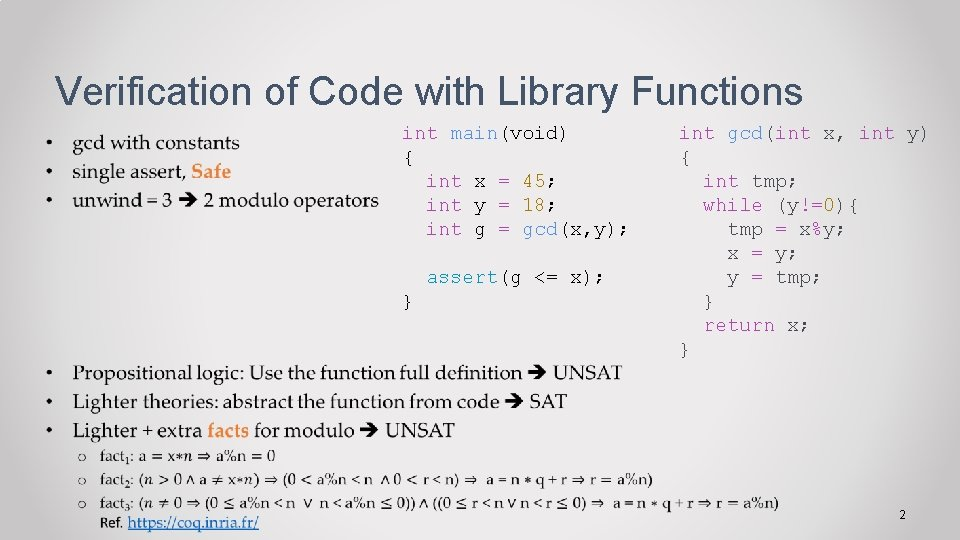Verification of Code with Library Functions • int main(void) { int x = 45; int y = 18; int g = gcd(x, y); assert(g <= x); } int gcd(int x, int y) { int tmp; while (y!=0){ tmp = x%y; x = y; y = tmp; } return x; } 2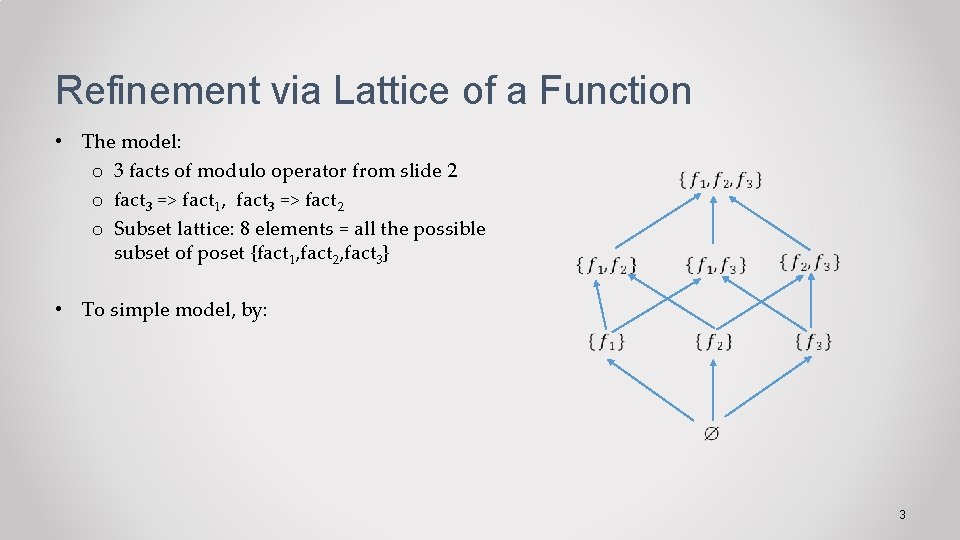Refinement via Lattice of a Function • The model: o 3 facts of modulo operator from slide 2 o fact 3 => fact 1, fact 3 => fact 2 o Subset lattice: 8 elements = all the possible subset of poset {fact 1, fact 2, fact 3} • To simple model, by: 3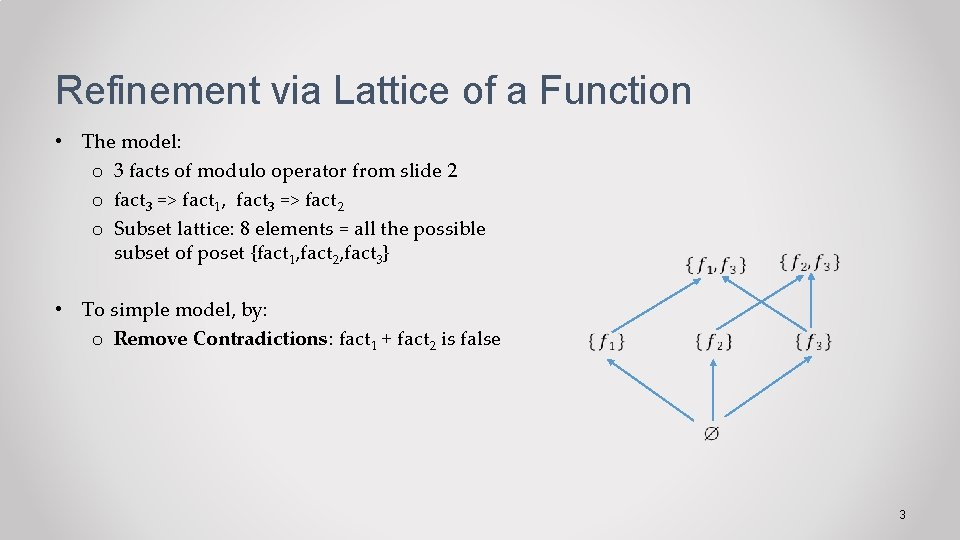Refinement via Lattice of a Function • The model: o 3 facts of modulo operator from slide 2 o fact 3 => fact 1, fact 3 => fact 2 o Subset lattice: 8 elements = all the possible subset of poset {fact 1, fact 2, fact 3} • To simple model, by: o Remove Contradictions: fact 1 + fact 2 is false 3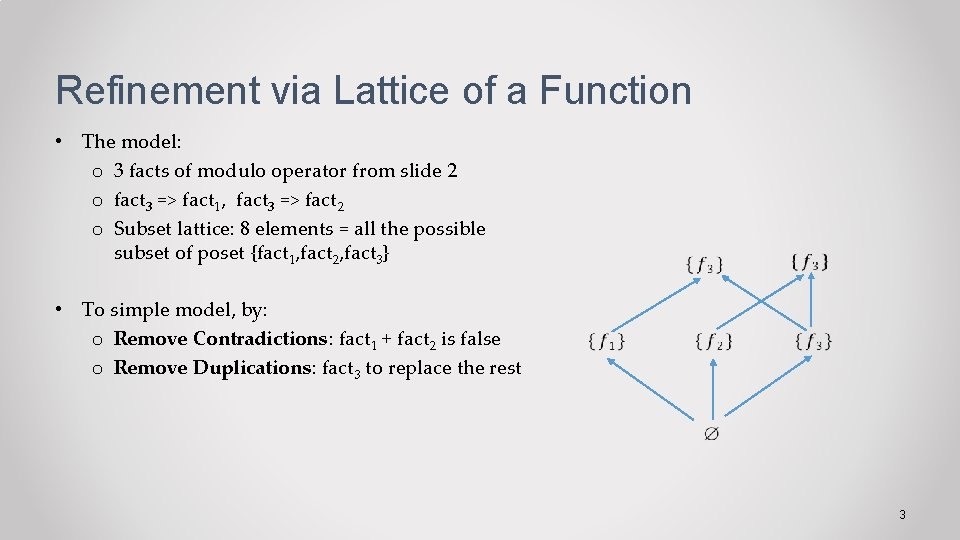Refinement via Lattice of a Function • The model: o 3 facts of modulo operator from slide 2 o fact 3 => fact 1, fact 3 => fact 2 o Subset lattice: 8 elements = all the possible subset of poset {fact 1, fact 2, fact 3} • To simple model, by: o Remove Contradictions: fact 1 + fact 2 is false o Remove Duplications: fact 3 to replace the rest 3Refinement via Lattice of a Function • The model: o 3 facts of modulo operator from slide 2 o fact 3 => fact 1, fact 3 => fact 2 o Subset lattice: 8 elements = all the possible subset of poset {fact 1, fact 2, fact 3} • To simple model, by: o Remove Contradictions: fact 1 + fact 2 is false o Remove Duplications: fact 3 to replace the rest 3Refinement via Lattice of a Function • The model: o 3 facts of modulo operator from slide 2 o fact 3 => fact 1, fact 3 => fact 2 o Subset lattice: 8 elements = all the possible subset of poset {fact 1, fact 2, fact 3} • To simple model, by: o Remove Contradictions: fact 1 + fact 2 is false o Remove Duplications: fact 3 to replace the rest • Output: New semilattice that is a reduced lattice of the original subset lattice 3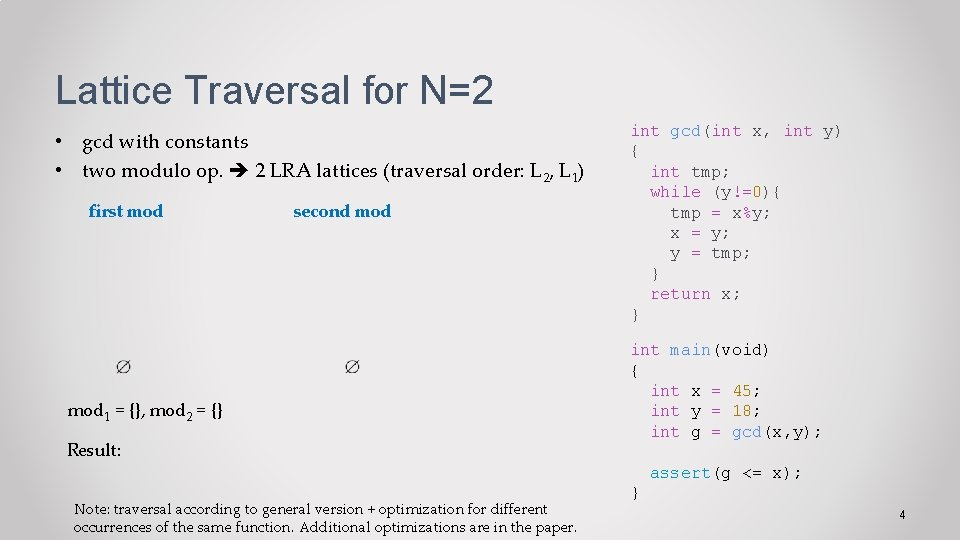Lattice Traversal for N=2 • gcd with constants • two modulo op. 2 LRA lattices (traversal order: L 2, L 1) first mod second mod 1 = {}, mod 2 = {} Result: Note: traversal according to general version + optimization for different occurrences of the same function. Additional optimizations are in the paper. int gcd(int x, int y) { int tmp; while (y!=0){ tmp = x%y; x = y; y = tmp; } return x; } int main(void) { int x = 45; int y = 18; int g = gcd(x, y); assert(g <= x); } 4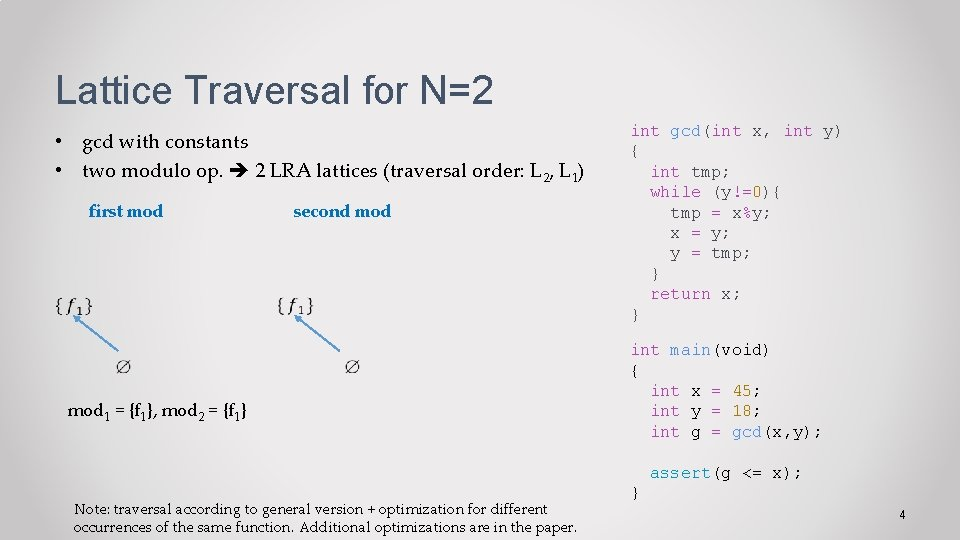Lattice Traversal for N=2 • gcd with constants • two modulo op. 2 LRA lattices (traversal order: L 2, L 1) first mod second mod 1 = {f 1}, mod 2 = {f 1} Note: traversal according to general version + optimization for different occurrences of the same function. Additional optimizations are in the paper. int gcd(int x, int y) { int tmp; while (y!=0){ tmp = x%y; x = y; y = tmp; } return x; } int main(void) { int x = 45; int y = 18; int g = gcd(x, y); assert(g <= x); } 4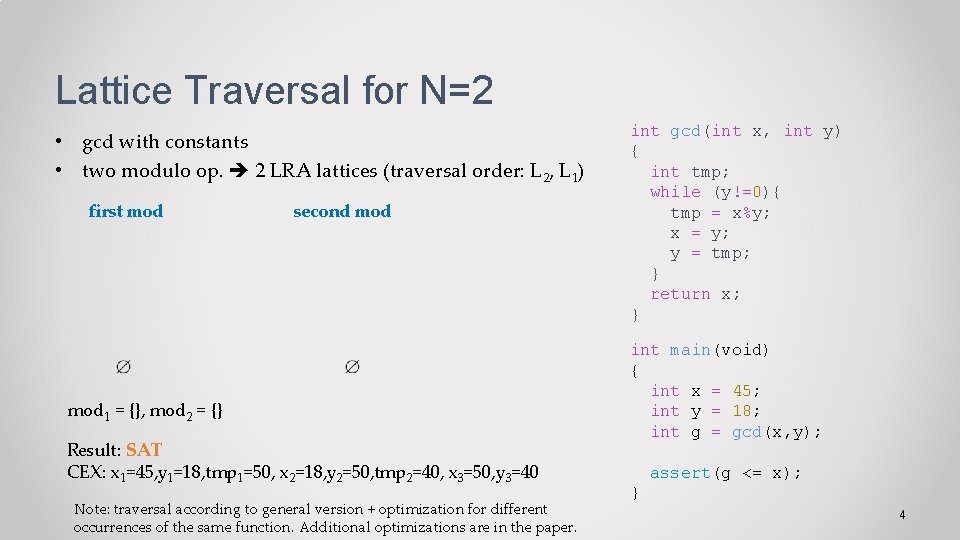Lattice Traversal for N=2 • gcd with constants • two modulo op. 2 LRA lattices (traversal order: L 2, L 1) first mod second mod 1 = {}, mod 2 = {} Result: SAT CEX: x 1=45, y 1=18, tmp 1=50, x 2=18, y 2=50, tmp 2=40, x 3=50, y 3=40 Note: traversal according to general version + optimization for different occurrences of the same function. Additional optimizations are in the paper. int gcd(int x, int y) { int tmp; while (y!=0){ tmp = x%y; x = y; y = tmp; } return x; } int main(void) { int x = 45; int y = 18; int g = gcd(x, y); assert(g <= x); } 4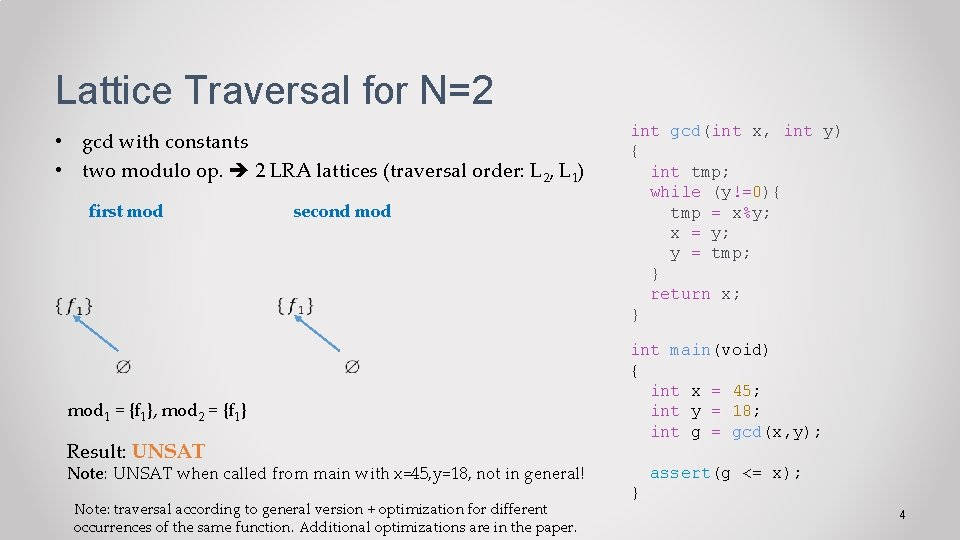Lattice Traversal for N=2 • gcd with constants • two modulo op. 2 LRA lattices (traversal order: L 2, L 1) first mod second mod 1 = {f 1}, mod 2 = {f 1} Result: UNSAT Note: UNSAT when called from main with x=45, y=18, not in general! Note: traversal according to general version + optimization for different occurrences of the same function. Additional optimizations are in the paper. int gcd(int x, int y) { int tmp; while (y!=0){ tmp = x%y; x = y; y = tmp; } return x; } int main(void) { int x = 45; int y = 18; int g = gcd(x, y); assert(g <= x); } 4Lattice Traversal for N=2 • gcd with constants • two modulo op. 2 LRA lattices (traversal order: L 2, L 1) first mod second mod 1 = {}, mod 2 = {f 1} Try now the other successor of ⊥ of L 1 (of operator mod 1) Note: traversal according to general version + optimization for different occurrences of the same function. Additional optimizations are in the paper. int gcd(int x, int y) { int tmp; while (y!=0){ tmp = x%y; x = y; y = tmp; } return x; } int main(void) { int x = 45; int y = 18; int g = gcd(x, y); assert(g <= x); } 4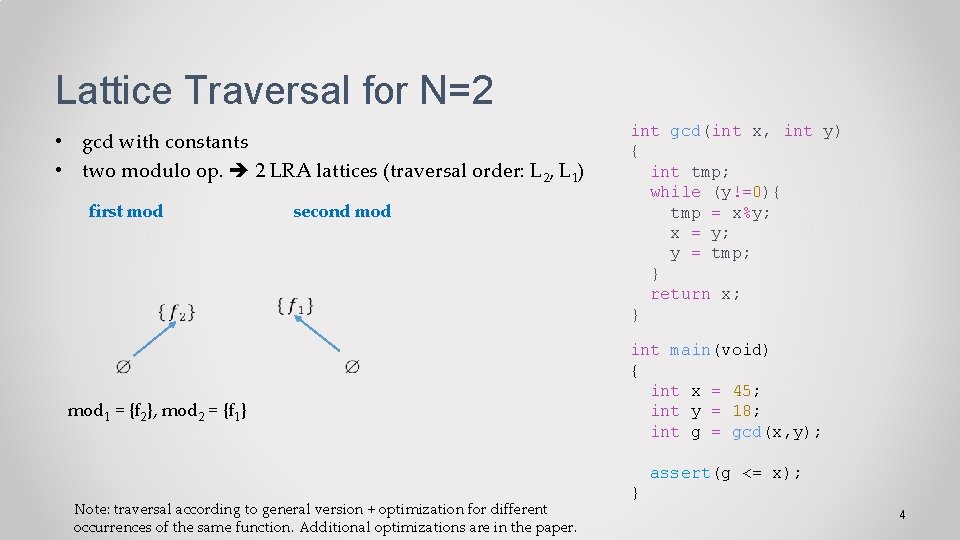Lattice Traversal for N=2 • gcd with constants • two modulo op. 2 LRA lattices (traversal order: L 2, L 1) first mod second mod 1 = {f 2}, mod 2 = {f 1} Note: traversal according to general version + optimization for different occurrences of the same function. Additional optimizations are in the paper. int gcd(int x, int y) { int tmp; while (y!=0){ tmp = x%y; x = y; y = tmp; } return x; } int main(void) { int x = 45; int y = 18; int g = gcd(x, y); assert(g <= x); } 4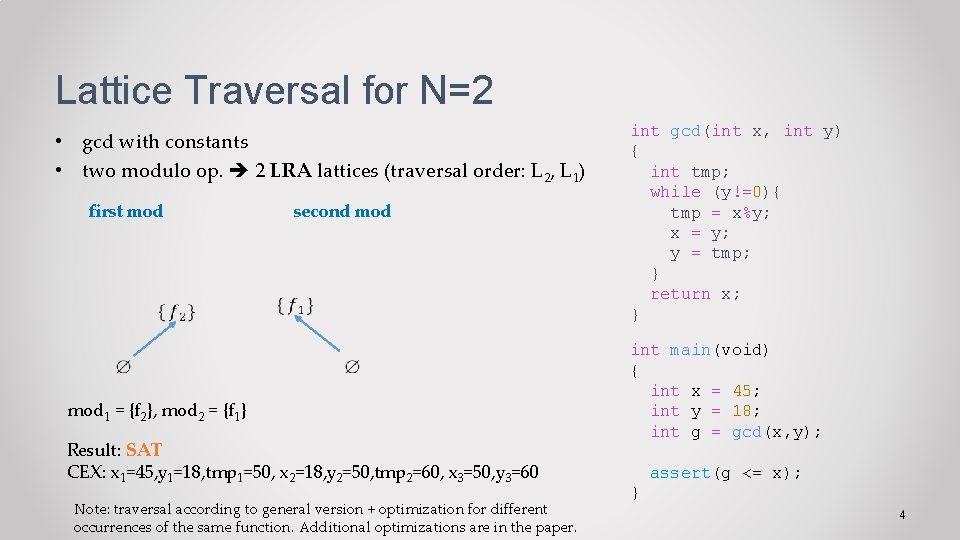Lattice Traversal for N=2 • gcd with constants • two modulo op. 2 LRA lattices (traversal order: L 2, L 1) first mod second mod 1 = {f 2}, mod 2 = {f 1} Result: SAT CEX: x 1=45, y 1=18, tmp 1=50, x 2=18, y 2=50, tmp 2=60, x 3=50, y 3=60 Note: traversal according to general version + optimization for different occurrences of the same function. Additional optimizations are in the paper. int gcd(int x, int y) { int tmp; while (y!=0){ tmp = x%y; x = y; y = tmp; } return x; } int main(void) { int x = 45; int y = 18; int g = gcd(x, y); assert(g <= x); } 4Lattice Traversal for N=2 • gcd with constants • two modulo op. 2 LRA lattices (traversal order: L 2, L 1) first mod second mod mod 1 = {f 3}, mod 2 = {f 3} Note: traversal according to general version + optimization for different occurrences of the same function. Additional optimizations are in the paper. int gcd(int x, int y) { int tmp; while (y!=0){ tmp = x%y; x = y; y = tmp; } return x; } int main(void) { int x = 45; int y = 18; int g = gcd(x, y); assert(g <= x); } 4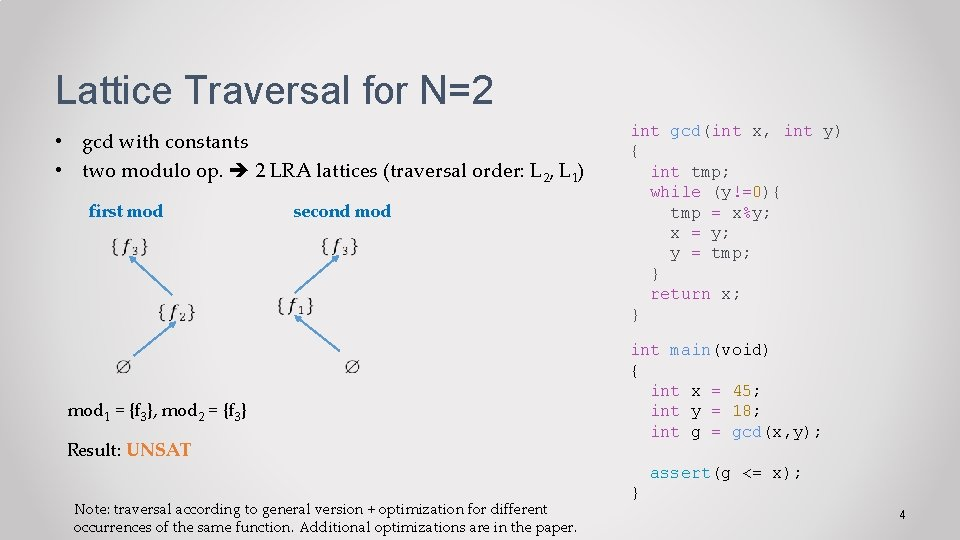Lattice Traversal for N=2 • gcd with constants • two modulo op. 2 LRA lattices (traversal order: L 2, L 1) first mod second mod mod 1 = {f 3}, mod 2 = {f 3} Result: UNSAT Note: traversal according to general version + optimization for different occurrences of the same function. Additional optimizations are in the paper. int gcd(int x, int y) { int tmp; while (y!=0){ tmp = x%y; x = y; y = tmp; } return x; } int main(void) { int x = 45; int y = 18; int g = gcd(x, y); assert(g <= x); } 4